Courses

# Test: Inventory Control (Level - 2)

## 20 Questions MCQ Test Manufacturing and Industrial Engineering | Test: Inventory Control (Level - 2)

Description
This mock test of Test: Inventory Control (Level - 2) for Mechanical Engineering helps you for every Mechanical Engineering entrance exam. This contains 20 Multiple Choice Questions for Mechanical Engineering Test: Inventory Control (Level - 2) (mcq) to study with solutions a complete question bank. The solved questions answers in this Test: Inventory Control (Level - 2) quiz give you a good mix of easy questions and tough questions. Mechanical Engineering students definitely take this Test: Inventory Control (Level - 2) exercise for a better result in the exam. You can find other Test: Inventory Control (Level - 2) extra questions, long questions & short questions for Mechanical Engineering on EduRev as well by searching above.
QUESTION: 1

### In a Wilsons model, annual demand is 10,000 units, the cost of placing an order is Rs. 500. If EOQ is 2000 units then minimum inventory cost per annum is

Solution:

D = 10,000 units

CO = Rs. 500/order

EOQ = 2000 units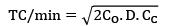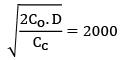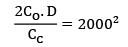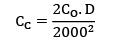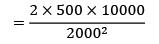CC = Rs. 25/unit/yr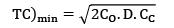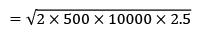= Rs. 5000

QUESTION: 2

Solution:
QUESTION: 3

### There are two products A and B with the following characteristics product demand (in units), order cost (in Rs./order), holding cost (in Rs./unit/years)A 100 100 4B 400 100 1The economic order quantities (EOQ) of product A and B will be in the ratio of

Solution:

EOQ of A and B is in ratio of 1:4 being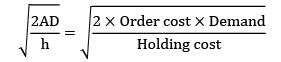QUESTION: 4

If the annual demand of an item becomes half, ordering cost double, holding cost one-fourth and the unit cost twice, then what is the ratio of the new EOQ and the earlier EOQ? [Assume that carrying cost depends on unit cost]

Solution: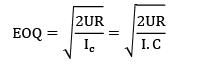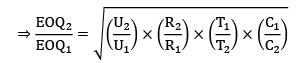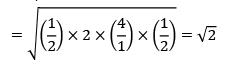QUESTION: 5

A shop owner with an annual constant demand of 'A' units has ordering costs of Rs. 'P' per order and carrying costs Rs. 'I' per unit per year. The economic order quantity for a purchasing model having no shortage may be determined from

Solution:
QUESTION: 6

If orders are placed once a month to meet an annual demand of 6,000 units, then the average inventory would be

Solution:

Number of orders ‘N’ =12

D = 6000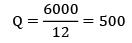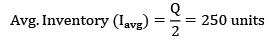*Answer can only contain numeric values
QUESTION: 7

The annual demand for an item is 12000 units and the ordering cost is Rs. 25/order. If the holding cost is 12% of the unit cost, then the number of orders per annum is __________ (Assume unit cost as Rs. 20)

Solution:

D = 12,000 units/year

Co = Rs. 25/order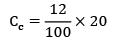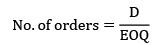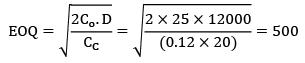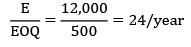*Answer can only contain numeric values
QUESTION: 8

If the orders are placed once in every two months to meet annual demand of 12,000 units. The carrying cost of inventory is Rs. 2/unit-year. What is the annual inventory cost (Rs)?

Solution: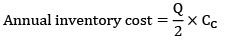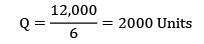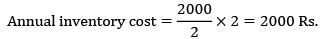QUESTION: 9

The demand for a commodity is 100 units per day. The ordering cost is Rs. 200/order and the carrying cost is Rs. 20/unit/year. If the lead time is 13 days, the re-order point is ____________

Solution:

Daily demand = 100 units

LT = 13 days

ROP/ROL = daily demand × LT

= 100 × 13 = 1300 units

*Answer can only contain numeric values
QUESTION: 10

The annual demand for a product is 60,000 units, the ordering cost is Rs. 8000 per order. Considering Wilsons model, the EOQ is 12,000

units. When the annual inventory cost is minimized, the annual inventory holding cost is Rs ___________

Solution:

D = 60,000

Co = Rs. 8000/order

EOQ = 12,000

Annual inventory holding cost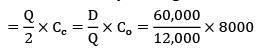= Rs. 40,000

QUESTION: 11

A company has an annual demand of 3000 units, the ordering cost per order is Rs. 50 and the carrying cost is Rs. 5 per unit-year. The backorder costs are estimated as Rs. 20 per unit-year. The maximum inventory is (approximately)

Solution:

D = 3000 units

Co = Rs. 50/order

Cc = Rs. 5/unit/year

Cs = Rs. 20/unit/year

Qs =?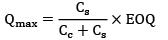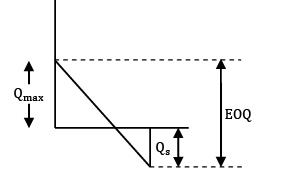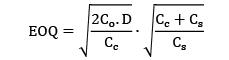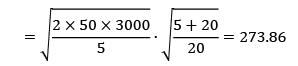≃ 274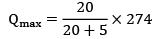Qmax = 219.2 ≈ 220

*Answer can only contain numeric values
QUESTION: 12

Televisions are produced in batches. The production rate is 80 units/day. The demand during production period is 50 units/day. The set-up cost of manufacturing process is 3000 per set-up and holding cost is Rs. 15/unit/year. What is the economic batch size?

Solution: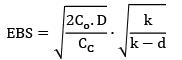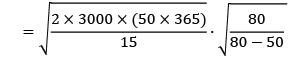= Rs. 4412

*Answer can only contain numeric values
QUESTION: 13

A manufacturing company has a contract to supply 5000 components to an automobile company per day. The manufacturing capacity of the company is 7000 components per day. The carrying cost of 1000 components is 20 paise per day. The set-up cost is Rs. 20. The total inventory cost per annum on optimum basis is __________

Solution:

Consumption rate = d = 5000/day

Production rate = k = 7000/day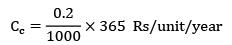= 0.073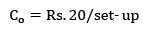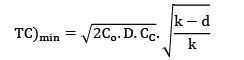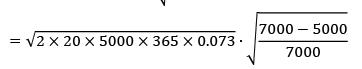TC)min = 1233.9

*Answer can only contain numeric values
QUESTION: 14

The annual demand for an item is 5200 units. The ordering cost is Rs. 200/order. The inventory holding cost on average inventory is 20%. The unit cost is Rs. 5 and the storage cost based on maximum inventory is 10 paise per unit per year. EOQ will be __________ units.

Solution: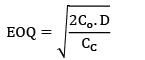Total CC = Holding cost + Storage cost

= 0.2 × 5 + 0.1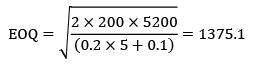*Answer can only contain numeric values
QUESTION: 15

A company follows an EOQ system with the following data

Annual demand = 15,000 units

unit cost = Rs. 50

ordering cost = Rs. 240/order

carrying cost = 12% × unit cost

safety stock = 150 units

The re-order level is __________ days.

(A)760

(B)770

Solution:

ROL = safety stock + Demand during lead time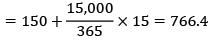QUESTION: 16

In an ideal inventory control system, the economic lot size for a part is 200 units. If the annual demand for the part is increased by 4 times and carrying cost is decreased by ½, the new EOQ is __________

Solution:

EOQ 1 = 200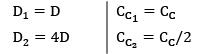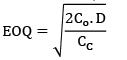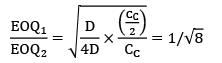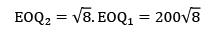*Answer can only contain numeric values
QUESTION: 17

The demand during lead time is 250 units. The annual consumption is 4000 units. The company has a policy of EOQ ordering and maintains a buffer stock of 200 units. The reorder level is _____________ units.

(A)450

(B)450

Solution:

ROL = safety stock + lead time demand

= 200 + 250

= 450

QUESTION: 18

Consider the following data:

Annual demand = 3000 units

Ordering cost = Rs. 100/order

Carrying cost = 25% of unit price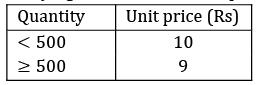Optimal order quantity is

Solution: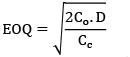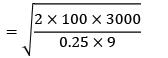EOQ = 516.39

(Satisfying the Range) Optimal order quantity = 516

*Answer can only contain numeric values
QUESTION: 19

A washer manufacturing company has a contract to supply 5000 washers to an automobile industry per day. The company has a capacity to manufacture 10,000 washers/day and holding cost of one washer is Rs. 0.2 per year. Set up cost is Rs. 20. The length of the production cycle is approximately (in days) __________.

Solution:

Consumption rate = d = 5000/day

production rate = k = 10000/day

Cc = Rs. 0.2/unit/year

Co = Rs. 20/order

Length of production cycle = cycle time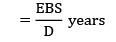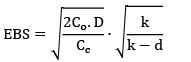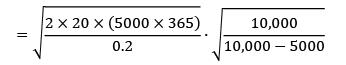= 27018.5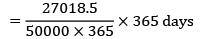= 5.4 days

QUESTION: 20

Annual consumption in ABC analysis can be represented as [D = annual demand, Q = ordering Quantity, Cu = unit cost]

Solution: